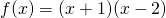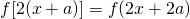# A different perspective to transformationOkay, many students get confused over when to do what for transformation. I thought I should try to clarify a bit. First of all, it doesn’t matter whether you translate or scale first! x and y transformations are independent so do them in whichever order you like. Most importantly, we need to understand that every single transformation gives a NEW function.

I’ll discuss a bit on how I help my students to do it safely.

So you can see it as a replacing “x” by “x+a” for example. The function f(x) becomes f(x+a) & ifthen it becomes. We literally replace the x by x+a. This is a translation in -a units along x axis.

If we extend this and consider replace x by 2x then we have. We apply the same method and it gives. This is a scaling by 1/2 units parallel to x axis.

Switch this around.

Consider instead we replace x by 2x first then x by x+a.

The functions will be f(x) becomes f(2x) and then. Here we scale by 1/2 units parallel to x axis than translate by -a units along the x axis. The resultant curve will be different from previous! Of course, we can also translate by -2a units along to x axis then scale by 1/2 units parallel to x axis, that is f(x) -> f(x+2a) -> f(2x+2a).

The act of replacing x by ___ is always consistent. But please don’t write it in this manner during exam; I’m merely suggesting an alternative way to do it correctly.

Consider something else, say f(-|x|).
For this we will replace x by -x first then replace x by |x|. Other words, we reflect about y-axis first then do |x|..
You should realise that replacing x by |x| then x by -x is pretty meaningless since we have. So the last transformation of -x is redundant.

Using this idea of replace x by ___, we in general do something I call like “outside in” if you want an easier brainless method.

Example:Starting we f(x), we go for a translation of 4units in along x axis, then a reflection about y axis then scale by 1/2 parallel to x axis then |x|. So really doing from furthest of x towards x, like outside in. You can try writing it out in function terms.

Students who need further clarification, feel free to discuss. I think it is a lot easier to understand this way. 🙂

Not readable? Change text.